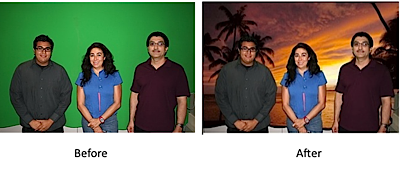# 1. Image Processing¶

Images can be seen as being a two-dimensional matrix of colors. In Java/Processing, this could be implemented as an array of arrays of colors. Or just a single array where the rows are listed one after another. We call this matrix of colors "pixels" short for "picture elements".

We alter an image by changing one pixel at at time; we can analyze a picture by accessing one pixel at a time. Both of these actions typically involve nested for-loops: one for width, and one for height. By doing this, we could:

• find objects in a picture
• do special effects, such as:
• obamicon - mapping all colors to 4 particular colors
• green screen effects, "chroma key compositing" -
• lighten an image
• edge detection
• any of a number of things, limited only by your creativity# 2. Image Processing in Processing¶

There are two ways of accessing a PImage's pixels in Processing:

1. one-by-one, using img.get(x, y) and img.set(x, y, c)
2. entire picture, using img.loadPixels(), use array, updatePixels()

## 2.1 One-by-one Pixel Access¶

Using Processing's PImage object, you can easily get/set a pixel's color by using the following interface:

### 2.1.1 Creating an PImage:¶

In [ ]:
PImage img = createImage(100, 100);
image(img, 0, 0);


### 2.1.2 Setting colors using get/set¶

In [ ]:
PImage img = createImage(100, 100);
for (int i=20; i < img.width; i++) {
for (int j=70; j < img.height; j++) {
img.set(i, j, color(255, 0, 0));
}
}
image(img, 0, 0);

In [ ]:
PImage img = createImage(100, 100);
for (int i=10; i < img.width - 10; i++) {
for (int j=10; j < img.height - 10; j++) {
img.set(i, j, color(0, 255, 0));
}
}
image(img, 0, 0);


## 2.2 Entire Picture Access¶

If you are going to access/change more than a few pixels, it is faster to:

1. convert the entire picture into an array using PImage.loadPixels()
2. access/change the array using PImage.pixels[POSITION]
3. if changed, convert the entire array into a image back to image using PImage.updatePixels()

### 2.2.1 Setting colors using an array¶

In [ ]:
PImage img = createImage(100, 100);
// Load colors from img into array:
// Access array:
for (int i=20; i < img.width; i++) {
for (int j=70; j < img.height; j++) {
img.pixels[i + (j * img.width)] = color(255, 0, 0);
}
}
// Load colors from array into img:
img.updatePixels();
image(img, 0, 0);

In [ ]:
PImage img = createImage(100, 100);
// Load colors from img into array:
// Access array:
for (int i=10; i < img.width-10; i++) {
for (int j=10; j < img.height-10; j++) {
img.pixels[i + (j * img.width)] = color(0, 255, 0);
}
}
// Load colors from array into img:
img.updatePixels();
image(img, 0, 0);


## 2.3 Assignment 8¶

For this assignment, you have a choice:

1. Do image processing on an image of your choice
2. Using image processing to find the orange tower

### 2.3.1 Find the Orange Tower¶

class MyRobot extends Robot {
MyRobot(float x, float y, float r) {
super(x, y, r);
}

void brain() {
PImage pic = this.takePicture();
// Show pic on canvas:
image(pic, 500 - 256, 250);
for (int i = 0; i < pic.width; i++) {
for (int j = 0; j < pic.height; j++) {
color pixel = pic.pixels[i + (j * pic.width)];
// ...
}
}
}
}

In :
%include Robot.pde
%include Hit.pde
%include World.pde

Robot robot;
World world;
int mx, my;

void mousePressed() {
mx = mouseX;
my = mouseY;
}

void mouseDragged() {
robot.forward((mouseX - mx)/100.0);
robot.turn(-(mouseY - my)/1000.0);
}

void mouseReleased() {
robot.stop();
}

class MyRobot extends Robot {
MyRobot(float x, float y, float r) {
super(x, y, r);
}

void brain() {
PImage pic = this.takePicture();
// Show pic on canvas:
image(pic, 500 - 256, 250);
for (int i = 0; i < pic.width; i++) {
for (int j = 0; j < pic.height; j++) {
color pixel = pic.pixels[i + (j * pic.width)];
// ...
}
}
}
}

void setup() {
// Set up canvas 500, 250 for World, 128 for camera:
size(500, 250 + 128);
// Set rate so it doesn't overwhelm your browser:
frameRate(10);
// Create a simulated world:
world = new World(500, 250);
// put orange tower in a random place:
int x = random(10, 400 - 25);
int y = random(10, 250 - 10 - 25);
world.addBox(x, y, x + 25, y + 25, color(255, 150, 0));
robot = new MyRobot(450, 125, 0);
}

void draw() {
// Clear:
background(255);
// Run simulation for one step:
world.update();
}

Sketch #50:

## 2.4 Bonus¶

Find the orange tower in a random, complex world:

In :
%include Robot.pde
%include Hit.pde
%include World.pde

Robot robot;
World world;
int mx, my;

void mousePressed() {
mx = mouseX;
my = mouseY;
}

void mouseDragged() {
robot.forward((mouseX - mx)/100.0);
robot.turn(-(mouseY - my)/1000.0);
}

void mouseReleased() {
robot.stop();
}

class MyRobot extends Robot {
MyRobot(float x, float y, float r) {
super(x, y, r);
}

void brain() {
PImage pic = this.takePicture();
// Show pic on canvas:
image(pic, 500 - 256, 250);
}
}

void setup() {
// Set up canvas 500, 250 for World, 128 for camera:
size(500, 250 + 128);
// Set rate so it doesn't overwhelm your browser:
frameRate(10);
// Create a simulated world:
world = new World(500, 250);

Integer[] colors = new Integer[] {color(255, 255, 0),
color(128, 0, 128),
color(255, 128, 255),
color(255, 0, 255),
};
for (int c = 1; c < 5; c++) {
int h = 100;
int y = random(10, 250 - h - 10);
world.addBox(100 * c, y, 100 * c + 10, y + h, colors[c]);
}
// put orange tower in a random place:
int x = random(10, 100 - 25);
int y = random(10, 250 - 10 - 25);
world.addBox(x, y, x + 25, y + 25, color(255, 128, 0));
// Create robot, and add to world:
robot = new MyRobot(450, 125, PI);